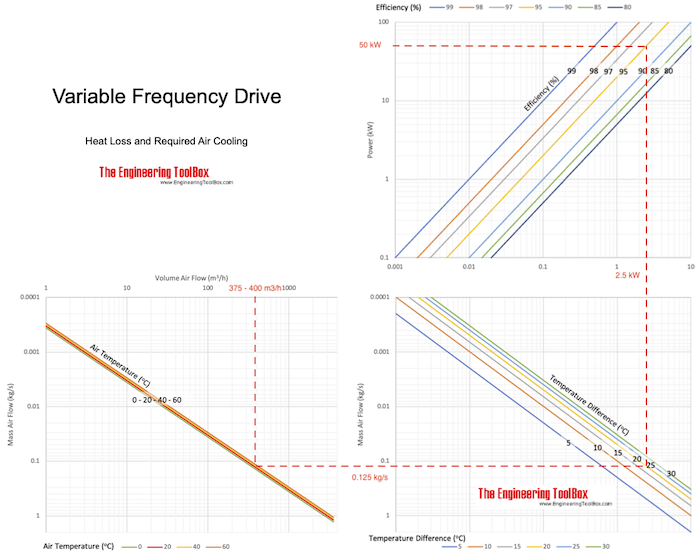Engineering ToolBox - Resources, Tools and Basic Information for Engineering and Design of Technical Applications!

# Variable Frequency Drives - Heat Loss and Required Air Cooling

## Avoid overheated variable frequency drives with adequate ventilation.

Variable frequency drives are common for controlling the electric motor speed in applications with fans, pumps, compressors, elevators, extruders etc.

### Heat Loss from a Variable Frequency Drive

An amount of the power transferred through a variable frequency drive to the motor is lost as heat. The heat loss from a drive can be expressed as

Hloss = Pt (1 - ηd)         (1)

where

Hloss = heat loss to the variable-frequency drive surroundings (kW)

Pt = electrical power through the variable-frequency drive (kW)

ηd = variable-frequency drive efficiency

The heat loss expressed in imperial units

Hloss = Pt 3412 (1 - ηd)         (1b)

where

Hloss = heat loss to the variable-frequency drive surroundings (btu/h)

Pt = power in to the frequency drive (kW)

ηd = variable-frequency drive efficiency

To calculate maximum heat loss - the maximum power transmission through the variable-frequency drive must be used.

It is common that the heat loss from a frequency drive is in the range 2 - 6% of the KVA rating.

### Necessary Ventilation for Cooling a Variable-Frequency Drive

Maximum ambient temperature for a frequency-drive is approximately 40oC (104oF). Since frequency-drives often are physical protected in small cabinets or small rooms, ventilation - or even cooling - may be needed to avoid overheating.

The mass flow of air needed for transporting heat from the variable-frequency drive can be expressed as

mair = Hloss / cp (tout - tin)         (2)

where

mair = mass flow of air (kg/s)

Hloss = heat loss to the frequency-drive surroundings (W)

cp = specific heat of air (kJ/kg oC) (1.005 kJ/kg oC standard air)

tout = temperature of air out (oC)

tin = temperature of air in (oC)

Combined with (1), the mass flow (2) can be expressed as:

mair = Pt (1 - ηd) / cp (tout - tin)         (2b)

The volume flow can be calculated by multiplying (2b) with the specific volume or inverted density:

qair = (1 / ρair) Pt (1 - ηd) / cp (tout - tin)         (2c)

where

ρair = density of air at the actual temperature (1.205 kg/m3 standard air)

#### Variable Frequency Drive - Heat Loss Air Cooling Chart#### Example - Ventilation and Cooling of a Variable-Frequency Drive

The heat generation by the variable frequency drive with maximum power of 100 kW and efficiency of 0.95,can be calculate with eq. 1 as

Hloss = (50 kW) (1 - 95 / 100)

= 2.5 kW

The mass of cooling cooling air through the cabinet when the maximum ambient operating temperature for the frequency drive is 40 oC and outside temperature of the cabinet is 20 oC can be calculated with eq. 2 as

mair = (2.5 kW) / (1.005 kJ/kg oC) ((40 oC) - (20 oC))

= 0.125 kg/s

The volume and density of air depends on the temperature of the air. The density of air at 20oC is 1.205 kg/m3 and 1.127 kg/m3 at 40 oC.

The volume flow at the inlet (20 oC):

qair = (1 / (1.205 kg/m3)) (0.125 kg/s)

= 0.104 m3/s

= 375 m3/h

The volume flow at the outlet (40 oC):

qair = (1 / (1.127 kg/m3)) (0.125 kg/s)

= 0.111 m3/s

= 400 m3/h

## Related Topics

• Electrical - Electrical units, amps and electrical wiring, wire gauge and AWG, electrical formulas and motors.
• Miscellaneous - Engineering related topics like Beaufort Wind Scale, CE-marking, drawing standards and more.

## Engineering ToolBox - SketchUp Extension - Online 3D modeling!

Add standard and customized parametric components - like flange beams, lumbers, piping, stairs and more - to your Sketchup model with the Engineering ToolBox - SketchUp Extension - enabled for use with the amazing, fun and free SketchUp Make and SketchUp Pro .Add the Engineering ToolBox extension to your SketchUp from the SketchUp Pro Sketchup Extension Warehouse!

Translate

## Privacy

We don't collect information from our users. Only emails and answers are saved in our archive. Cookies are only used in the browser to improve user experience.

Some of our calculators and applications let you save application data to your local computer. These applications will - due to browser restrictions - send data between your browser and our server. We don't save this data.

## Citation

• Engineering ToolBox, (2004). Variable Frequency Drives - Heat Loss and Required Air Cooling. [online] Available at: https://www.engineeringtoolbox.com/variable-frequency-drives-d_656.html [Accessed Day Mo. Year].

Modify access date.

. .

#### Scientific Online Calculator6 24